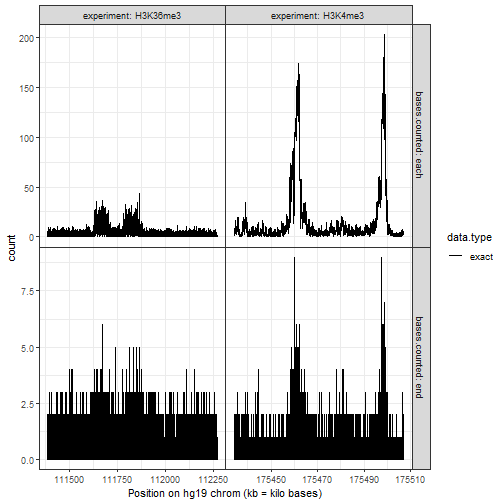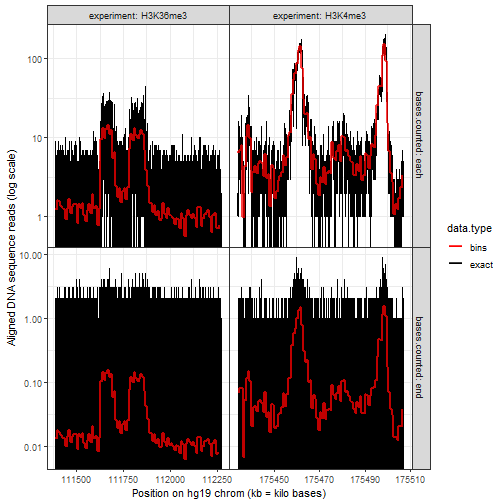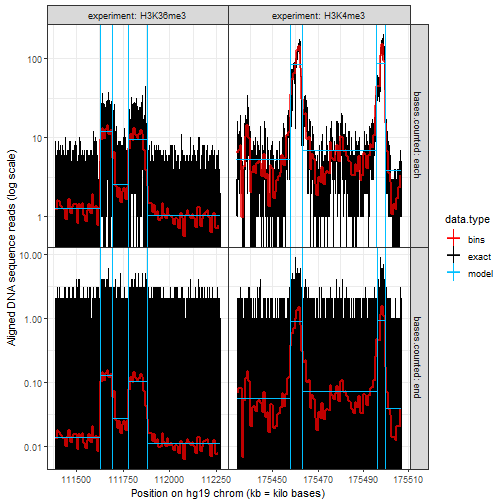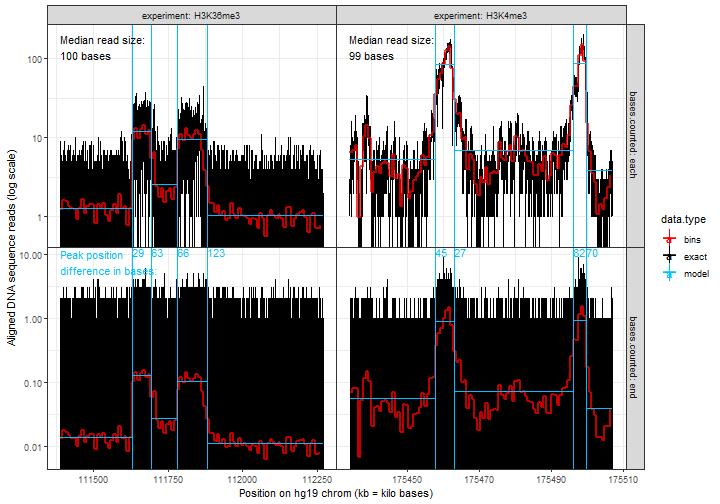In this vignette we explore to what extent the peak predictions are sensitive to the spatial correlation in typical genomic data. We also demonstrate how to efficiently compute coverage profiles from raw aligned read data using the data.table package.

First we load a data set with one row for every genomic region with a unique aligned read, and compute mean read size in bases.

library(data.table)
(experiments <- ChIPreads[, .(
mean.bases=mean(chromEnd-chromStart),
median.bases=median(chromEnd-chromStart),
chromStart=min(chromStart)
), by=list(experiment)])
#>    experiment mean.bases median.bases chromStart
#> 1:   H3K36me3   93.88568          100  111387372
#> 2:    H3K4me3   95.36224           99  175434087

From the table above it is clear that the average read size is about 100 for these two experiments.

Below, for each of the two data sets, we compute a data table with two representations of these aligned reads: count each read at each aligned base in the read (this induces spatial correlation), or just the end/last base of the read (this has no spatial correlation).

end.counts <- ChIPreads[, list(
count=.N #ignores dup reads, sum(count) would not.
), by=list(experiment, chrom, chromEnd)]
(aligned.dt <- rbind(
bases.counted="each", experiment, chrom,
chromStart, chromEnd,
count=1)], #ignore duplicate reads.
end.counts[, .(
bases.counted="end", experiment, chrom,
chromStart=chromEnd-1L, chromEnd,
count)]))
#>        bases.counted experiment chrom chromStart  chromEnd count
#>     1:          each   H3K36me3  chr9  111387372 111387472     1
#>     2:          each   H3K36me3  chr9  111387436 111387536     1
#>     3:          each   H3K36me3  chr9  111387443 111387543     1
#>     4:          each   H3K36me3  chr9  111387455 111387554     1
#>     5:          each   H3K36me3  chr9  111387566 111387666     1
#>    ---
#> 74841:           end    H3K4me3  chr2  175506898 175506899     1
#> 74842:           end    H3K4me3  chr2  175506912 175506913     1
#> 74843:           end    H3K4me3  chr2  175506943 175506944     1
#> 74844:           end    H3K4me3  chr2  175506956 175506957     1
#> 74845:           end    H3K4me3  chr2  175507001 175507002     1

Each row of the data table above describes how one read should be counted in order to compute an aligned read profile.

aligned.dt[, {
as.list(quantile(chromEnd-chromStart))
}, by=.(bases.counted, experiment)]
#>    bases.counted experiment 0% 25% 50% 75% 100%
#> 1:          each   H3K36me3 20  98 100 100  115
#> 2:          each    H3K4me3 20  97  99 100  107
#> 3:           end   H3K36me3  1   1   1   1    1
#> 4:           end    H3K4me3  1   1   1   1    1

The table above shows that when we only count the end of each base, we only count the read at one position. When we count the read at each position, that means from 20 to 115 bases, depending on the read.

Next we compute a count profile for each base in these genomic regions.

(seq.dt <- aligned.dt[, {
event.dt <- rbind(
data.table(count, pos=chromStart+1L),
data.table(count=-count, pos=chromEnd+1L))
edge.vec <- event.dt[, {
as.integer(seq(min(pos), max(pos), l=100))
}]
event.bins <- rbind(
event.dt,
data.table(count=0L, pos=edge.vec))
total.dt <- event.bins[, .(
count=sum(count)
), by=list(pos)][order(pos)]
total.dt[, cum := cumsum(count)]
total.dt[, bin.i := cumsum(pos %in% edge.vec)]
## it is somewhat confusing because total.dt pos is the first base
## with cum, and cum goes all the way up to but not including the
## pos of the next row.
total.dt[, data.table(
chromStart=pos[-.N]-1L,
chromEnd=pos[-1]-1L,
count=cum[-.N],
bin.i=bin.i[-.N])]
}, by=list(bases.counted, experiment, chrom)])
#>         bases.counted experiment chrom chromStart  chromEnd count bin.i
#>      1:          each   H3K36me3  chr9  111387372 111387436     1     1
#>      2:          each   H3K36me3  chr9  111387436 111387443     2     1
#>      3:          each   H3K36me3  chr9  111387443 111387455     3     1
#>      4:          each   H3K36me3  chr9  111387455 111387472     4     1
#>      5:          each   H3K36me3  chr9  111387472 111387536     3     1
#>     ---
#> 124619:           end    H3K4me3  chr2  175506943 175506944     1    99
#> 124620:           end    H3K4me3  chr2  175506944 175506956     0    99
#> 124621:           end    H3K4me3  chr2  175506956 175506957     1    99
#> 124622:           end    H3K4me3  chr2  175506957 175507001     0    99
#> 124623:           end    H3K4me3  chr2  175507001 175507002     1    99

In contrast to the previous data tables, the table above contains no information about individual reads. Each row represents how many reads, count, have been counted at all positions between chromStart+1 and chromEnd. We plot these aligned read counts below:

library(ggplot2)
gg.data <- ggplot()+
theme_bw()+
theme(panel.spacing=grid::unit(0, "lines"))+
facet_grid(
bases.counted ~ experiment,
scales="free",
labeller=label_both)+
geom_step(aes(
chromStart/1e3, count, color=data.type),
data=data.table(seq.dt, data.type="exact"))+
scale_color_manual(values=c(
exact="black",
bins="red",
model="deepskyblue"
))+
scale_x_continuous("Position on hg19 chrom (kb = kilo bases)")
print(gg.data)It is clear from the plot above that, for each experiment (left:H3K36, right:H3K4), the profiles in the top and bottom plots have peaks in similar regions.

Next we compute mean profile in bins, using the bin.i column we created earlier, which assigns each genomic region to one of 99 bins.

bin.dt <- seq.dt[, {
bases <- chromEnd - chromStart
data.table(
binStart=min(chromStart),
binEnd=max(chromEnd),
mean.count=sum(count*bases)/sum(bases),
bases=sum(bases)
)}, by=list(bases.counted, experiment, bin.i)]
gg.bins <- gg.data+
geom_step(aes(
binStart/1e3, mean.count, color=data.type),
alpha=0.75,
size=1,
data=data.table(bin.dt, data.type="bins"))+
scale_y_log10("Aligned DNA sequence reads (log scale)")
print(gg.bins)
#> Warning: Transformation introduced infinite values in continuous y-axisThe mean counts in each bin appear in the plot above as a red line.

Next we compute the optimal segmentation model with 2 peaks for each data set.

if(interactive() && requireNamespace("future"))future::plan("multiprocess")
segs.dt <- seq.dt[, {
data.dir <- file.path(tempdir(), bases.counted, experiment)
dir.create(data.dir, showWarnings=FALSE, recursive=TRUE)
coverage.bedGraph <- file.path(data.dir, "coverage.bedGraph")
fwrite(
.SD[, .(chrom, chromStart, chromEnd, count)],
coverage.bedGraph,
sep="\t",
quote=FALSE,
col.names=FALSE)
fit <- PeakSegDisk::sequentialSearch_dir(data.dir, 2L, verbose=1)
data.table(fit\$segments, data.type="model")
}, by=list(bases.counted, experiment)]
#> Next = 0, Inf
#> Next = 110.889323103247
#> Next = 1385.23387063487
#> Next = 25425.6282786644
#> Next = 373396.954452715
#> Next = 116338.388376185
#> Next = 0, Inf
#> Next = 192.185946075722
#> Next = 11247.0775923531
#> Next = 176157.439626319
#> Next = 0, Inf
#> Next = 4.37603211086448
#> Next = 8.94288335356839
#> Next = 32.2910962473304
#> Next = 839.262095714024
#> Next = 0, Inf
#> Next = 4.20444060519522
#> Next = 20.44214129621
#> Next = 618.412457529431
changes.dt <- segs.dt[, {
.SD[-1]
}, by=list(bases.counted, experiment, data.type)]
gg.model <- gg.bins+
geom_segment(aes(
chromStart/1e3, mean,
xend=chromEnd/1e3, yend=mean,
color=data.type),
data=segs.dt)+
geom_vline(aes(
xintercept=chromEnd/1e3,
color=data.type),
data=changes.dt)
print(gg.model)
#> Warning: Transformation introduced infinite values in continuous y-axisThe plot above shows that the predicted peaks (blue) of the models occur in similar positions, using either the each or end representations of the data.

Next we compute the difference between predicted peak positions:

peaks.dt <- segs.dt[status=="peak"]
peaks.dt[, peak.i := rep(1:2, l=.N)]
peak.pos.tall <- melt(
peaks.dt,
measure.vars=c("chromStart", "chromEnd"))
peak.pos.wide <- dcast(
peak.pos.tall,
experiment + variable + peak.i ~ bases.counted)
peak.pos.wide[, diff.bases := abs(each-end)]
bases.max.dt <- seq.dt[, .(max.count=max(count)), by=list(bases.counted)]
diff.panel <- "end"
diff.y <- bases.max.dt[
diff.panel, max.count, on=list(bases.counted)]
diff.y <- Inf
diff.vjust <- 1.1
gg.model+
geom_text(aes(
"Median read size:\n%.0f bases",
median.bases)),
hjust=0,
vjust=1,
geom_text(aes(
end/1e3, diff.y,
label=diff.bases,
color=data.type),
data=data.table(
bases.counted=diff.panel,
data.type="model",
peak.pos.wide),
vjust=diff.vjust,
hjust=0)+
geom_text(aes(
chromStart/1e3, diff.y,
label="Peak position\ndifference in bases:",
color=data.type,
),
hjust=0,
vjust=diff.vjust,
data=data.table(
data.type="model",
bases.counted=diff.panel,
experiments["H3K36me3", on=list(experiment)]))
#> Warning: Transformation introduced infinite values in continuous y-axisIn the plot above the blue numbers show the differences (in bases) between the top and bottom panel peak predictions. It is clear that the peak predictions are highly consistent between the each/end representations, with variation on the order of read size (100 bases).

Overall these results indicate that the peak detection algorithm is highly robust to the spatial correlation that is present in typical ChIP-seq coverage profile data.